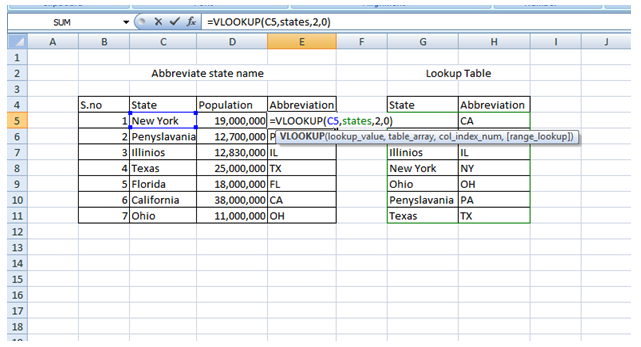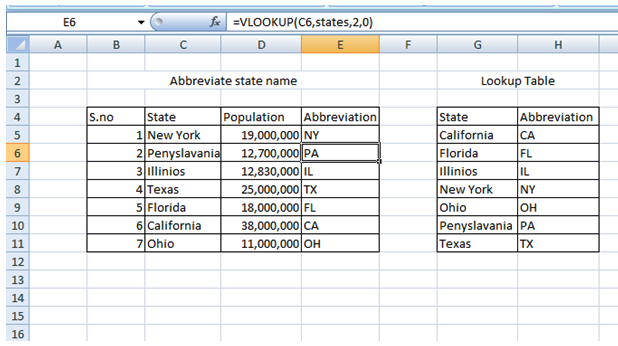Get instant live expert help with Excel or Google Sheets“My Excelchat expert helped me in less than 20 minutes, saving me what would have been 5 hours of work!”

#### Post your problem and you'll get expert help in seconds

Your message must be at least 40 characters
Our professional experts are available now. Your privacy is guaranteed.

# How to Abbreviate State Names in Excel

Many times, we need to write abbreviations of names, places, and things. This can be done easily by using inbuilt functions of Excel. In order to determine two-letter abbreviations from full state names (i.e., Los Angeles to LA), you can use a simple formula using VLOOKUP.

## VLOOKUP function to abbreviate state names in Excel

VLOOKUP is an Excel function that is used to look up and retrieve data from a specific lookup column in a table. It is also categorized as a lookup/reference function.

### Syntax of VLOOKUP

`=VLOOKUP(value, table,index number,[range_lookup])`

In this case, there is no direct way for you to finish this job in Excel. But, you can list all the state names and their relative abbreviations first in a table and then apply the VLOOKUP function to get any of the required abbreviations.

In this case, we’ll use the formula stated below:

### Generic formula

`=VLOOKUP(name,states,2,0)`

### Example

In the example shown, we create a lookup table with two columns where we list all the names of the states in one column and their relative abbreviations in another. Then, we create another table consisting of three columns where we mention state name in one column, their population in second and in the third column, we’ll get their abbreviation displayed.

We apply the formula in E5

`=VLOOKUP(C5,states,2,0)`

Here, ‘states’ is a named range/lookup table G5:H11Figure 1: Illustration of the VLOOKUP formula

Once we apply this formula, we’ll get the abbreviation NW for New York in E5. Similarly, on dragging the formula to other cells, we’ll get the abbreviation of other states as shown.Figure 2: VLOOKUP for abbreviating state name

VLOOKUP will locate the matching entry in the “states” table and in return, it will give the corresponding two-letter abbreviations in column E.

Still need some help with Excel formatting or have other questions about Excel? Connect with a live Excel expert here for some 1 on 1 help. Your first session is always free.

### Did this post not answer your question? Get a solution from connecting with the expert.Another blog reader asked this question today on Excelchat:## Subscribe to Excelchat.coAnother blog reader asked this question today on Excelchat: